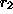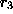Next: The chr (Constraint Handling Up: Complex Constraints Previous: Complex Constraints

## The propia (Generalised Propagation) Library

A major issue in defining complex constraints is how to handle disjunction. The resource constraint of our running example can be quite easily expressed using a disjunction of finite domain constraints. Indeed ECLiPSe allows us to express disjunction as alternative clauses defining a predicate, so the constraint can be expressed as a single ECLiPSe predicate thus:

```fdTaskResource(S1,E1,R1,S2,E2,R2) :-
R1 ## R2.
R1#=R2, S1 #>= E2.
R1#=R2, S2 #>= E1.```

The purpose of the propia library is to take exactly such disjunctive definitions and turn them into constraints!

This is illustrated below.

```:- lib(propia).

propiaTR(S1,R1,S2,R2) :-
[S1,S2]::0..100, [R1,R2]::[r1,r2,r3],
E1 = S1+50,  E2 = S2+70,

R1 ## R2.
R1#=R2, S1 #>= E2.
R1#=R2, S2 #>= E1.```
Specifying a Resource Contention Constraint in ECLiPSe
The syntax Goal infers most turns any ECLiPSe goal into a constraint. It is supported by the propia library.

The behaviour of this constraint is to find which values for each variable are consistent with the constraint. The constraint has the propagation behaviour described in [Wal97]: it repeatedly attempts to reduce the domains of its variables further every time any other constraints reduce any of these domains. figureshows some examples of this behaviour. In query 2, the constraint deduces that, since the tasks cannot overlap, taskcannot start between 51 and 69, and taskcannot start between 31 and 49. In query 3, since the tasks are bound to overlap, the constraint deduces that taskmust use either resourceor.

```[eclipse 1]: [fdTaskResource].
*     yes.

[eclipse 2]: propiaTR(S1, R1, S2, R2), R1#=r1, R2#=r1.
*     S1 = S1{[0..50, 70..100]}
*     R1 = r1,
*     S2 = S2{[0..30, 50..100]},
*     R2 = r1
*     yes.

[eclipse 3]: propiaTR(S1,R1,S2,R2), R1=r1, S2#>=35, S2#<=45.
*     S1 = S1{[0..100]}
*     R1 = r1,
*     S2 = S2{[35..45]},
*     R2 = R2{[r2, r3]}
*     yes.```
The Behaviour of Goal infers most

Other behaviour can be achieved by writing Goal infers consistent or Goal infers ground instead. These behaviours, together with other facilities of the propia library are described in the ECLiPSe extensions manual [Be97].

Joachim Schimpf
Wed Sep 3 18:07:19 BST 1997